• 已经可以通过这些来写一些简单的编程题目了，同时也是巩固自己知识的时候啦！ 以下编程题目是从GitHub上找到的一个很管用的学习资源，推荐新入门的小伙伴关注骆昊大神，因为他的教程超级nice！能够帮你少走很多...
    前面已经学习了Python中最基本的语法：变量、类型、运算符、表达式、分支结构、循环结构等。已经可以通过这些来写一些简单的编程题目了，同时也是巩固自己知识的时候啦！

以下编程题目是从GitHub上找到的一个很管用的学习资源，推荐新入门的小伙伴关注骆昊大神，因为他的教程超级nice！能够帮你少走很多弯路！

python运算符及其使用与input函数

华氏温度转换为摄氏温度（提示：华氏温度到摄氏温度的转换公式为：C=(F - 32) /1.8）

#F_C.py

'''
练习1：华氏温度转换为摄氏温度
提示：华氏温度到摄氏温度的转换公式为：$C=(F - 32) \div 1.8$。
'''

#提示用户输入华氏温度,并转化为对应的数值
f=float(input("请输入华氏温度："))
#print(f)

#对温度进行转化
c=(f-32)/1.8

'''
数据的输出
错误输出：print("当前华氏温度:%f,对应的摄氏温度为：%f",f,c)
Python中数据的输入与输出
输入：input函数
输出：print函数，使用占位符语法：百分号代替逗号，输出的变量要用括号
'''
print("当前华氏温度:%.2f,对应的摄氏温度为：%f" % (f,c))

输入圆的半径计算计算周长和面积。

#CirculOperate.py

'''
编程练习：输入圆的半径计算计算周长和面积
时间：2020年1月13日11:05:13
作者：Dragon
'''

r=float(input("请输入圆的半径："))
pi=3.14;
s=2*pi*r;
area=pi*r*r;
print("半径为",r,'的周长为:',s,' 面积为：',area)


输入年份判断是不是闰年。

#IsRunYear.py

'''
编程练习：输入年份判断是不是闰年,如果是闰年输出True 否则输出False
时间：2020年1月13日11:10:46
作者：Dragon
提示：能被4且不能被100或者400整除的数
'''

year=int(input("please input your year："))
if (year % 4 == 0 and year % 100 != 0) or \
year % 400 == 0:
print(year,"True")
else:
print(year,"False")



分支结构 if-else语句的使用，elif与else

用户身份验证

#UserValid.py

'''
编程练习 if-else语句
描述：用户身份验证
正确的账号 admin 和密码 0000
验证成功输出：welcome admin!
验证失败输出：
a.user name is fail
b.password is fail
加强版：循环验证，直到验证正确
作者：Dragon
'''

username=input("please input your username:")
if (username!="admin"):
print("user name is fail")
else:
password=input("please input your password:")
if password!="0000":
print("password is fail")
else:
print("welcome admin!")

分段函数求值

3x - 5  (x > 1)
分段函数f(x) =  x + 2   (-1 <= x <= 1)
5x + 3  (x < -1)

#PiecewiseFunction.py
'''
编程实战：分段函数求值
3x - 5  (x > 1)
分段函数f(x) =  x + 2   (-1 <= x <= 1)
5x + 3  (x < -1)
时间：2020年1月13日11:34:11
作者：Dragon
'''

x=float(input("请输入你的数值："))
if x<-1:
f=5*x+3;
elif x<=1:
f=x+2;
else:
f=3*x-5;
print("对应的结果为：",f)

百分制成绩转换为等级制成绩：
如果输入的成绩在90分以上（含90分）输出A；
80分-90分（不含90分）输出B；
70分-80分（不含80分）输出C；
60分-70分（不含70分）输出D；
60分以下输出E。

#ScoreToLevel.py

'''
编程练习：百分制成绩转换为等级制成绩：
如果输入的成绩在90分以上（含90分）输出A；
80分-90分（不含90分）输出B；
70分-80分（不含80分）输出C；
60分-70分（不含70分）输出D；
60分以下输出E。
时间：2020年1月13日11:40:06
作者：Dragon
'''

score=float(input("请输入您的成绩："))
if score<60:
print("成绩为E，您要再多多努力！")
elif score<70:
print("成绩为D，您要再多多努力！")
elif score<80:
print("成绩为c，加油！")
elif score<90:
print("成绩为B，不错哦~")
else:
print("成绩为A，学霸啊！")

循环结构：for-in循环，一种是while循环，range函数的使用三种常见的方式

用for循环实现1~100求和

#AddByFor.py

'''
用for循环实现1~100求和
时间：2020年1月14日10:54:22
作者：Dragon
注意for-in的语法格式
range的三种使用方法：
range(101)可以产生一个0到100的整数序列。
range(1, 100)可以产生一个1到99的整数序列。
range(1, 100, 2)可以产生一个1到99的奇数序列，其中2是步长，即数值序列的增量。
'''

sum=0;
for x in range(0,101):
sum+=x
print(sum)

猜数字游戏
计算机出一个1~100之间的随机数由人来猜
计算机根据人猜的数字分别给出提示大一点/小一点/猜对了
注意导入import random，random.randint（）函数的使用

#GuessNumber.py
import random   #导入random

'''
描述：计算机出一个1~100之间的随机数由人来猜
计算机根据人猜的数字分别给出提示大一点/小一点/猜对了
时间：2020年1月14日11:01:36
作者：Dragon
注意：生成随机数需要导入import random，random.randint（）函数的使用
'''

#系统生成1-100随机数字
randomNumber=random.randint(1,100)
#print("我是作弊器：随机数是：",randomNumber)

#提示用户输入数字
while True:
userNumber=int(input("请输入您的数字："))
if(userNumber<randomNumber):
print("再大一点！")
elif userNumber>randomNumber:
print("再小一点！")
else:
print("猜对了")
break


输出乘法口诀表(九九表)

#PrintMulTable.py

'''
描述:输出乘法口诀表(九九表)
时间：2020年1月14日11:11:19
作者：Dragon
思路：先思考一下乘法表长什么样，该怎么输出！
一行一行输出，列成递增
行：1-9
列：1-9
输出格式：a*b=c,不换行输出且制表
考虑完毕，动手！
'''

for row in range(1,10):
for cow in range(1,10):
if cow <= row:
print("%d * %d = %d \t" %(cow,row,row*cow),end="")
#print("%d * %d = %d " %(cow,row,row*cow),end="\t"),两种方式皆可
else:
break
print()     #换行


输入一个正整数判断是不是素数

#IsPrime.py

'''
描述：
时间：2020年1月14日11:28:48
作者：Dragon
思路：素数指的是只能被1和自身整除的大于1的整数
通过循环看其有没有其他因子
'''

number=int(input("请输入要判断的数字："))
flag=0;
for x in range(2,number):
#print("进入循环，当前x为：",x)
#print("number%x==0   为：",number/x==0)
if(number%x==0):
print("不是素数！")
flag=1
break

if flag==0:
print("是素数！")


打印如下所示的三角形图案。


*
**
***
****
*****



*
**
***
****
*****



*
***
*****
*******
*********



'''
描述：打印三角形图案。
时间：2020年1月14日12:17:02
作者：Dragon
'''

'''
打印第一种图案
*
**
***
****
*****
'''
for r1 in range(1,6):
for c1 in range(1,6):
if c1<=r1:
print("*",end="")
print()

'''
打印第二种图案
*
**
***
****
*****
'''
for r2 in range(1,6):
for c2 in range(1,6):
if (6-c2) > r2:
print(" ",end="")
else:
print("*", end="")
print()

'''
打印第三种图案：1,3,5,7,9
*    2i-1个，n行，【2n-(2i-1)】/2
***
*****
*******
*********
'''
for i in range(5):
for _ in range(5 - i - 1):
print(' ', end='')
for _ in range(2 * i + 1):
print('*', end='')
print()


展开全文• Pycharm题目来源：PTA编程实例1：日期格式化世界上不同国家有不同写日期习惯。比如美国人习惯写成“月-日-年”，而中国人习惯写成“年-月-日”。下面请你写个程序，自动把读入美国格式日期改写成中国习惯...
环境：Win10操作系统；Python3.7；Pycharm题目来源：PTA编程实例1：日期格式化世界上不同国家有不同的写日期的习惯。比如美国人习惯写成“月-日-年”，而中国人习惯写成“年-月-日”。下面请你写个程序，自动把读入的美国格式的日期改写成中国习惯的日期。输入格式：输入在一行中按照“mm-dd-yyyy”的格式给出月、日、年。题目保证给出的日期是1900年元旦至今合法的日期。输出格式：在一行中按照“yyyy-mm-dd”的格式给出年、月、日。输入样例：03-15-2017输出样例：2017-03-15str =input()mm= str.split("-",2)dd= str.split("-",2)yy= str.split("-",2)date= yy+"-"+mm+"-"+ddprint(date)split()方法：str.split(str="",num=string.count(str)).str -- 分隔符，默认为所有的空字符，包括空格、换行(
)、制表符(	)等。num -- 分割次数。默认为 -1, 即分隔所有。编程实例2：整数四则运算本题要求编写程序，计算2个正整数的和、差、积、商并输出。题目保证输入和输出全部在整型范围内。输入格式:输入在一行中给出2个正整数A和B。输出格式:在4行中按照格式“A 运算符 B = 结果”顺序输出和、差、积、商。输入样例:3 2输出样例:3 + 2 = 53 - 2 = 13 * 2 = 63 / 2 = 1方法1：a,b =map(int,input().split())print("{} + {} = {}".format(a,b,a+b))print("{} + {} = {}".format(a,b,a-b))print("{} * {} = {}".format(a,b,a*b))print("{} / {} = {}".format(a,b,a//b))方法2：A,B =map(int, input().split())c=str(A)d=str(B)print(c+"+"+d+"=",A+B);print(c+"-"+d+"=",A-B);print(c+"*"+d+"=",A*B);print(c+"/"+d+"=",A//B);map()方法：会根据提供的函数对指定序列做映射。第一个参数 function 以参数序列中的每一个元素调用 function 函数，返回包含每次 function 函数返回值的新列表。map(function,iterable,...)function -- 函数iterable -- 一个或多个序列format方法：Python2.6 开始，新增了一种格式化字符串的函数 str.format()，它增强了字符串格式化的功能。基本语法是通过 {} 和 : 来代替以前的 % 。format 函数可以接受不限个参数，位置可以不按顺序。举个栗子：print("{}{}".format("hello", "world"))  #一一对应，输出 hello  worldprint("{0}{1}".format("hello", "world")) #输出 hello  worldprint("{1}{0}".format("hello", "world")) #设置指定位置，输出 world  helloprint("{0}{0}{1}".format("hello", "world")) #设置指定位置，输出 hello  hello  worldformat另一个常用用法是分割字符串print('{:.2f}'.format(11.25555)) #表示保留两位小数，输出11.26print('{:.2%}'.format(0.1125555)) #表示转化为保留两位小数的百分位数，输出11.26%编程实例3：计算各对应位乘积之和读入两个整数a和b，输出绝对值a和绝对值b的各对应位乘积之和，如a=1234，b=608，则输出值为：“1×0+2×6+3×0+4×8“的值，即44。输入格式:在一行中输入两个数输出格式:在一行中输出对应位乘积之和输入样例:在这里给出一组输入。例如：1234 608输出样例:在这里给出相应的输出。例如：44a,b =map(int,input().split())a=abs(a)b=abs(b)a=str(a)b=str(b)sum=0if len(a)>len(b):for i inrange(0,len(b)):sum= sum + int(a[i+len(a)-len(b)])*int(b[i])if len(a)sum= sum + int(b[i+len(b)-len(a)])*int(a[i])if len(a)==len(b):for i inrange(0,len(a)):sum= sum + int(a[i])*int(b[i])print(sum)编程实例4：求出歌手的得分输入一个正整数n (n>4)，再输入n个实数，求出歌手的得分（保留2位小数）。设一歌唱评奖晚会上有n(n>4)个评委为歌手打分.评分规则:每个评委依次打分,再去掉2个最高分和2个最低分,计算余下的分数平均值为歌手的得分.输入格式:在第一行中输入n 在第二行中输入n个分数输出格式:在一行中输出平均分数输入样例:在这里给出一组输入。例如：1010 10 9 9 9 8 8 8 7 7输出样例:在这里给出相应的输出。例如：aver=8.50n=input()num= [int(n) for n ininput().split()]num.sort()for i in range(0,4):num.pop()num.reverse()sum=0for j inrange(len(num)):sum+=num[j]aver= sum/len(num)print("{:.2f}".format(aver))importnumpy as npn=input()num= [int(n) for n ininput().split()]num.sort()for i in range(0,4):num.pop()num.reverse()sum=0for j inrange(len(num)):sum+=num[j]#可以使用numpy自带的均值函数avg =np.mean(num)print("{:.2f}".format(avg))reverse()方法：将表中的元素反向存储pop()方法：Python 字典 pop() 方法删除字典给定键 key 及对应的值，返回值为被删除的值。key 值必须给出。 否则，返回 default 值。pop(key[,default])key: 要删除的键值default: 如果没有 key，返回 default 值注意1：在调用pop函数后，会对原来的列表产生影响，也就是说，pop函数删除的是原列表的值举个栗子：num = [1,2,3,4,5]num.pop()print(num)#输出[1, 2, 3, 4]注意2：pop函数里加入不同的值结果是不同的，默认列表中的元素首位标号为0，末尾元素为-1，依次类推举个栗子：num = [1,2,3,4,5]num.pop(-1)print(num)#输出[1, 2, 3, 4]num.pop(0)print(num)#输出[2, 3, 4]编程实例5：删除字符输入一个字符串 str，再输入要删除字符 c，大小写不区分，将字符串 str 中出现的所有字符 c 删除。输入格式:在第一行中输入一行字符 在第二行输入待删除的字符输出格式:在一行中输出删除后的字符串输入样例:在这里给出一组输入。例如：BeeE输出样例:在这里给出相应的输出。例如：result: Bstr =list(input().strip())x=input().strip()s= [i for i in str if not(i.lower() == x or i.upper() ==x)]print(s)print('result: %s' %''.join(s).strip())strip()方法：Python strip() 方法用于移除字符串头尾指定的字符（默认为空格或换行符）或字符序列。注意：该方法只能删除开头或是结尾的字符，不能删除中间部分的字符。str.strip([chars]);chars -- 移除字符串头尾指定的字符序列。举个栗子：str = "123abcrunoob321"print (str.strip( '12' ))  #字符序列为 12,输出3abcrunoob3str1 = "123456"print(str1.strip())  #输出123456,无首位空格lower()方法与upper()方法：Python lower() 方法转换字符串中所有大写字符为小写。 python upper()方法转换字符串中所有小写字符为大写举个栗子：str = "hello!"print (str.upper())  #输出HELLO!str1 = "HELLO!"print(str1.lower())  #输出hello!编程实例6：jmu-python-统计成绩输入一批学生成绩，计算平均成绩，并统计不及格学生人数。输入格式:每行输入一个数据，输入数据为负数结束输入输出格式:平均分=XX,不及格人数=XX,其中XX表示对应数据。如果没有学生数据，输出没有学生输入样例:305070809020-1输出样例:平均分=56.67,不及格人数=3a =float(input())list=[]sum=alist.append(a)count= 1if a >=0:while 1:a=float(input())if a <0:breaklist.append(a)sum= a +sumcount= count + 1print("平均分={:.2f},不及格人数=".format(sum / count), end="")n=0for i inlist:if i < (sum /count):n= n + 1print("%d" %n)else:print("没有学生")编程实例7：jmu-python-重复元素判定每一个列表中只要有一个元素出现两次，那么该列表即被判定为包含重复元素。编写函数判定列表中是否包含重复元素，如果包含返回True，否则返回False。然后使用该函数对n行字符串进行处理。最后统计包含重复元素的行数与不包含重复元素的行数。输入格式:输入n，代表接下来要输入n行字符串。然后输入n行字符串，字符串之间的元素以空格相分隔。输出格式:True=包含重复元素的行数， False=不包含重复元素的行数,后面有空格。输入样例:51 2 3 4 51 3 2 5 41 2 3 6 11 2 3 2 11 1 1 1 1输出样例:True=3, False=2n =int(input())f=0t=0for i inrange(n):a=input()a=list(a.split())if len(list(a)) ==len(set(a)):f+= 1else:t+= 1print('True=%d, False=%d' %(t,f))set()方法：set() 函数创建一个无序不重复元素集，可进行关系测试，删除重复数据，还可以计算交集、差集、并集等。举个栗子：x = set('aaabbc')print(x)  #输出{'a', 'c', 'b'}y = set('abcddee')print(y)   #输出{'d', 'c', 'b', 'a', 'e'}print(set(x&y))  #输出{'a', 'b', 'c'}print(set(x|y)) #输出{'d', 'a', 'c', 'b', 'e'}print(set(y-x)) #输出{'d', 'e'}
展开全文• 注意：我用的python2.7，大家如果用Python3.0以上版本，请记得在print()函数哦！如果因为版本问题评论，不做回复哦！！21.题目：将一个正整数分解质因数。例如：输入90,打印出90=2*3*3*5。程序分析：对n进行分解...
注意：我用的python2.7，大家如果用Python3.0以上的版本，请记得在print()函数哦！如果因为版本问题评论的，不做回复哦！！21.题目：将一个正整数分解质因数。例如：输入90,打印出90=2*3*3*5。程序分析：对n进行分解质因数，应先找到一个最小的质数k，然后按下述步骤完成：(1)如果这个质数恰等于n，则说明分解质因数的过程已经结束，打印出即可。(2)如果n!=k，但n能被k整除，则应打印出k的值，并用n除以k的商,作为新的正整数你n,重复执行第一步。(3)如果n不能被k整除，则用k+1作为k的值,重复执行第一步。注意：要知道 format 这个函数，其实就是格式化，与 print 差不多，具体可参考https://www.jb51.net/article/63672.htm方法一：只用循环# -*- coding: UTF-8 -*-while True:n = input("请输入一个数:")#这里有逗号是为了保证它输出后不自动换行，下同print '{} = '.format(n),#判断你输入的是不是正确格式if not isinstance(n, int) or n <= 0 :print '请输入一个正确的数字 !'#如果你是输入的是 1，那只输出 1if n in  :print '{}'.format(n)while n >= 2:for i in range(2,n + 1):if n % i == 0:n /= i  #n 等于 n/iif n == 1:print i ,else :  # index 一定是素数print '{} *'.format(i),breakprint  #换行咯方法二：定义函数# -*- coding: UTF-8 -*-def reduceNum(n):print '{} = '.format(n),if not isinstance(n, int) or n <= 0 :print '请输入一个正确的数字 !'exit(0)elif n in  :print '{}'.format(n)while n not in  : # 循环保证递归for i in range(2, n + 1) :if n % i == 0:n /= i # n 等于 n/iif n == 1:print ielse : # i 一定是素数print '{} *'.format(i),breakreduceNum(100)reduceNum(9)输出：100 = 2 * 2 * 5 * 59 = 3 * 3方法三：用列表 append 函数，这里只是用列表的形式输出了所有x的因子# -*- coding: UTF-8 -*-while True:x = input("pls input a number:")ret = []while x > 1:for v in range(2,x + 1):if x % v == 0:ret.append(v)x /= vbreakprint ret输出：pls input a number:9[3, 3]说明：其实两种方法大同小异，没什么区别。22.题目：求s=a+aa+aaa+aaaa+aa…a的值，其中a是一个数字。例如2+22+222+2222+22222(此时共有5个数相加)，几个数相加有键盘控制。程序分析：关键是计算出每一项# -*- coding: UTF-8 -*-Tn = 0Sn = [] #用来存储数字个数n = int(raw_input('输入你想要相加的数字个数 n = :')) #控制你要相加的次数a = int(raw_input('输入数字 a = :')) #真正要加的数字for count in range(n):Tn = Tn + aa = a * 10Sn.append(Tn)#每加一个数就放在Sn列表中print Tn#lambda这个函数比较强大，具体用法可以看下资料Sn = reduce(lambda x,y : x + y,Sn)print Sn输入你想要相加的数字个数 n = :5输入数字 a = :666666666666666674070用到的函数：1.python中的reduce内建函数是一个二元操作函数，他用来将一个数据集合（链表，元组等）中的所有数据进行下列操作：用传给reduce中的函数 func()（必须是一个二元操作函数）先对集合中的第1，2个数据进行操作，得到的结果再与第三个数据用func()函数运算，最后得到一个结果。23.题目：一个数如果恰好等于它的因子之和，这个数就称为”完数”。例如6=1＋2＋3.编程找出1000以内的所有完数。程序分析：请参照程序Python 练习实例14。只要在后面加上判断条件就可以了，注意，要在输出因子的时候加上1，注意看题，1是任何数的因子，所以不能漏掉# -*- coding: UTF-8 -*-import randomimport timewhile True:x = random.randint(1,1000)#因为后面求质数要修改x的值，所以先把x保存起来备用s = xfactors =  #1是任何数的因子while x > 1:#上限取值可以是x，即因子也可以是x本身，这个大家都懂得for v in range(2,x + 1):if x % v == 0:factors.append(v)#找到了当前的最小因子，重置继续找下一符合条件的最小因子x /= v#由于break是跳出当前的循环，所以这里的break是跳出for循环breakprint "所有因子是 %s,所以"%(factors),if sum(factors) == s: #这里就是判断函数了print "%d 是完数"%(s)else:print "%d 不是完数"%(s)time.sleep(1) #为防止程序卡顿，我睡一秒输出一个输出：所有因子是 [1, 2, 2, 2, 31],所以: 248 不是完数所有因子是 [1, 2, 2, 2, 73],所以: 584 不是完数所有因子是 [1, 823],所以: 823 不是完数所有因子是 [1, 281],所以: 281 不是完数所有因子是 [1, 467],所以: 467 不是完数所有因子是 [1, 2, 359],所以: 718 不是完数所有因子是 [1, 2, 7, 47],所以: 658 不是完数所有因子是 [1, 751],所以: 751 不是完数所有因子是 [1, 3, 71],所以: 213 不是完数24.题目：两个乒乓球队进行比赛，各出三人。甲队为a,b,c三人，乙队为x,y,z三人。已抽签决定比赛名单。有人向队员打听比赛的名单。a说他不和x比，c说他不和x,z比，请编程序找出三队赛手的名单。程序分析：用数学的角度很好理解是吧，但是这里要把字母转化成ASCII码来做哦！# -*- coding: UTF-8 -*-for i in range(ord('x'),ord('z') + 1):for j in range(ord('x'),ord('z') + 1):if i != j:for k in range(ord('x'),ord('z') + 1):if (i != k) and (j != k):if (i != ord('x')) and (k != ord('x')) and (k != ord('z')):print 'order is a -- %s	 b -- %s	c--%s' % (chr(i),chr(j),chr(k))25.题目：有一分数序列：2/1，3/2，5/3，8/5，13/8，21/13…求出这个数列的前20项之和。程序分析：请抓住分子与分母的变化规律。可以看出，分母是斐波那契数列哦~方法一：第一个分数的分子分母之和是第二个数的分子。# -*- coding: UTF-8 -*-a = 2.0  #第一个数的分母b = 1.0  #第一个数的分子s = 0   #前20项的和for n in range(1,21):s += a / bt = a  #先把分子保存到ta = a + b #分子是前一个分数的分子分母之和b = t  #分子分母交换print s方法二：# -*- coding: UTF-8 -*-a = 2.0b = 1.0s = 0.0for n in range(1,21):s += a / bb,a = a , a + bprint s方法三：# -*- coding: UTF-8 -*-a = 2.0b = 1.0l = []for n in range(1,21):b,a = a,a + bl.append(a / b)print reduce(lambda x,y: x + y,l)26.题目：求1+2!+3!+…+20!的和。程序分析：此程序只是把累加变成了累乘。想一想阶乘怎么算的？n!=n×(n-1)×(n-2)×(n-3)×…×1，所以只需要一个遍历，把n以内的数字相乘后，再加起来即可。方法一：# -*- coding: UTF-8 -*-n = 0s = 0t = 1for n in range(1,21):t *= n #这里t就是20以内每个数的阶乘s += t #把每次得到的阶乘值加到sprint '1! + 2! + 3! + ... + 20! = %d' % s方法三：这里用定义函数的方法，使用sum函数。# -*- coding: UTF-8 -*-s = 0l = range(1,21)def op(x):r = 1for i in range(1,x + 1):r *= ireturn rs = sum(map(op,l))print '1! + 2! + 3! + ... + 20! = %d' % s输出：1! + 2! + 3! + ... + 20! = 2561327494111820313这里补充一下map()函数的用法：很简单，第一个参数接收一个函数名，第二个参数接收一个可迭代对象# -*- coding: UTF-8 -*-ls = [1,2,3]rs = map(str, ls)#打印结果 ['1', '2', '3']lt = [1, 2, 3, 4, 5, 6]def add(num):return num + 1rs = map(add, lt)print rs#打印结果[2,3,4,5,6,7]27.题目：利用递归方法求5!。程序分析：递归公式：fn=fn_1*4!递归的方法就是自己调用自己。# -*- coding: UTF-8 -*-def jiecheng(n):if n <= 1:return 1else:return n * jiecheng(n-1)print jiecheng(5)输出：12028.题目：将所输入的5个字符，以相反顺序打印出来。方法一：利用递归函数调用方式# -*- coding: UTF-8 -*-def output(s,l):if l==0:returnprint (s[l-1])output(s,l-1)s = raw_input('Input a string:')l = len(s)output(s,l)输出：Input a string:12344321方法二：用reverse函数# -*- coding: UTF-8 -*-a = [1,2,3,4,5]a.reverse()print a或者：# -*- coding: UTF-8 -*-s = list(raw_input('Input a string:'))s.reverse()print s28.题目：有5个人坐在一起，问第五个人多少岁？他说比第4个人大2岁。问第4个人岁数，他说比第3个人大2岁。问第三个人，又说比第2人大两岁。问第2个人，说比第一个人大两岁。最后问第一个人，他说是10岁。请问第五个人多大？程序分析：利用递归的方法，递归分为回推和递推两个阶段。要想知道第五个人岁数，需知道第四人的岁数，依次类推，推到第一人（10岁），再往回推。方法一：这个很简单吧，就是每次循环都加2，循环4次就好了啊！# -*- coding: UTF-8 -*-x = 10for i in range(1,5):x = x + 2print x方法二：定义函数的方法# -*- coding: UTF-8 -*-def age(n):if n == 1:x = 10else:x = age(n - 1) + 2return xprint age(5)29.题目：一个5位数，判断它是不是回文数。即12321是回文数，个位与万位相同，十位与千位相同。程序分析：将这个五位数从中间分开，根据对称性去切，然后比较是否相等。# -*- coding: UTF-8 -*-a = int(raw_input("请输入一个数字:"))x = str(a)flag = Truefor i in range(len(x)/2):if x[i] != x[-i - 1]: #如果不相等，直接跳出循环，直接判断不是回文数flag = Falsebreakif flag:print "%d 是一个回文数!" % aelse:print "%d 不是一个回文数!" % a输出：请输入一个数字:1565115651 是一个回文数!30.题目：请输入星期几的第一个字母来判断一下是星期几，如果第一个字母一样，则继续判断第二个字母。程序分析：用情况语句比较好，如果第一个字母一样，则判断用情况语句或if语句判断第二个字母。。哇，这个题应该好简单啊~~先看一下星期的单词都有哪些“Monday Tuesday Wednesday Thursday Friday Saturday Sunday”，所有只有“T”和“S”要判断第二个字母。# -*- coding: UTF-8 -*-letter = raw_input("please input:")#while letter != 'Y':if letter == 'S':print ('please input second letter:')letter = raw_input("please input:")if letter == 'a':print ('Saturday')elif letter == 'u':print ('Sunday')else:print ('data error')elif letter == 'F':print ('Friday')elif letter == 'M':print ('Monday')elif letter == 'T':print ('please input second letter')letter = raw_input("please input:")if letter == 'u':print ('Tuesday')elif letter == 'h':print ('Thursday')else:print ('data error')elif letter == 'W':print ('Wednesday')else:print ('data error')这篇文章就介绍到这了，后面脚本之家小编将继续为大家分享更多的关于python的一些实例资料。
展开全文• 目录简易编程题目一、从键盘接收一百分制成绩 （0-100）， 要求输出其对应成绩等级 A-E。 其中，90 分以上为 'A' ， 80-89分为 'B' ， 70~79 分为'C' ， 60-69 分为 'D' ， 60 分以下为 'E' 。二、用python编写...
简易编程题目
目录简易编程题目一、从键盘接收一百分制成绩 （0-100）， 要求输出其对应的成绩等级 A-E。 其中，90 分以上为 'A' ， 80-89分为 'B' ， 70~79 分为'C' ， 60-69 分为 'D' ， 60 分以下为 'E' 。二、用python编写程序，输入一年份，判断该年份是否是闰年并输出结果三、预设一个0-9之间的整数，让用户猜一猜并输入所猜的数，如果大于预设的数，显示“太大”，小于预设的数，显示“太小"，如此循环，直至猜中该数，显示“恭喜 你猜中了”四、求1-100间所有偶数的和五、输入三个数，比较大小输出最大的数总结
一、从键盘接收一百分制成绩 （0-100）， 要求输出其对应的成绩等级 A-E。 其中，90 分以上为 ‘A’ ， 80-89分为 ‘B’ ， 70~79 分为’C’ ， 60-69 分为 ‘D’ ， 60 分以下为 ‘E’ 。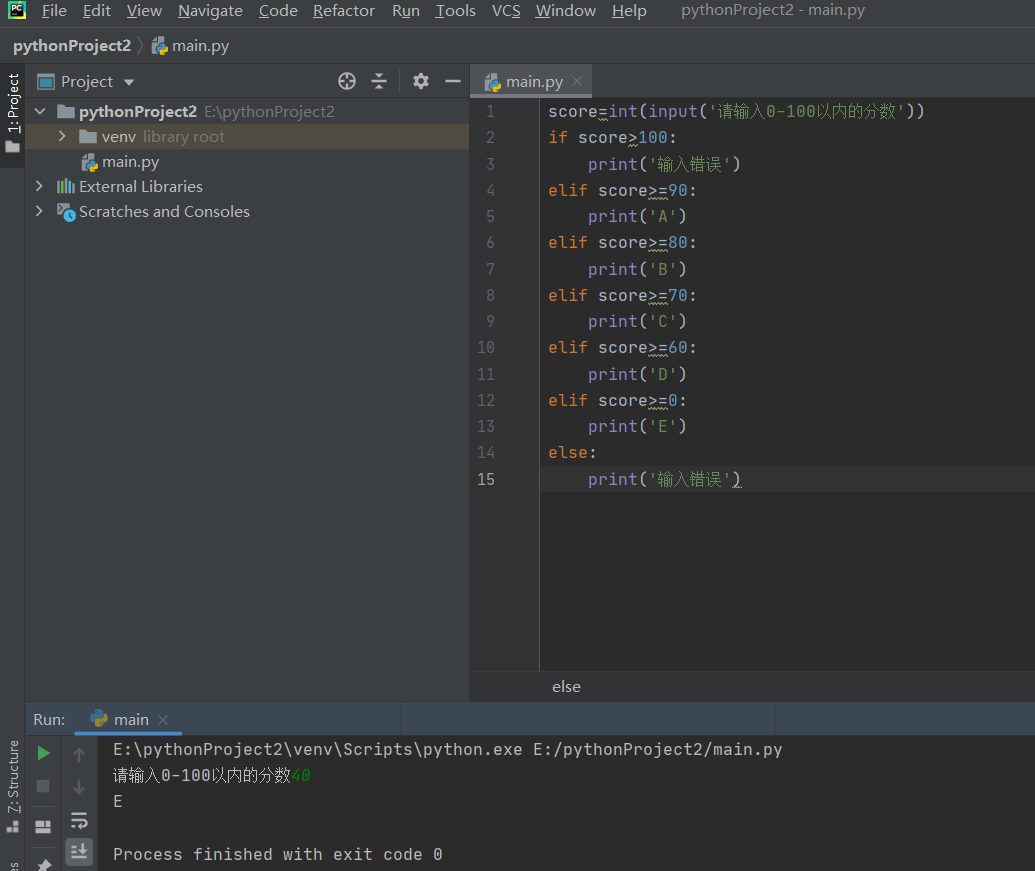二、用python编写程序，输入一年份，判断该年份是否是闰年并输出结果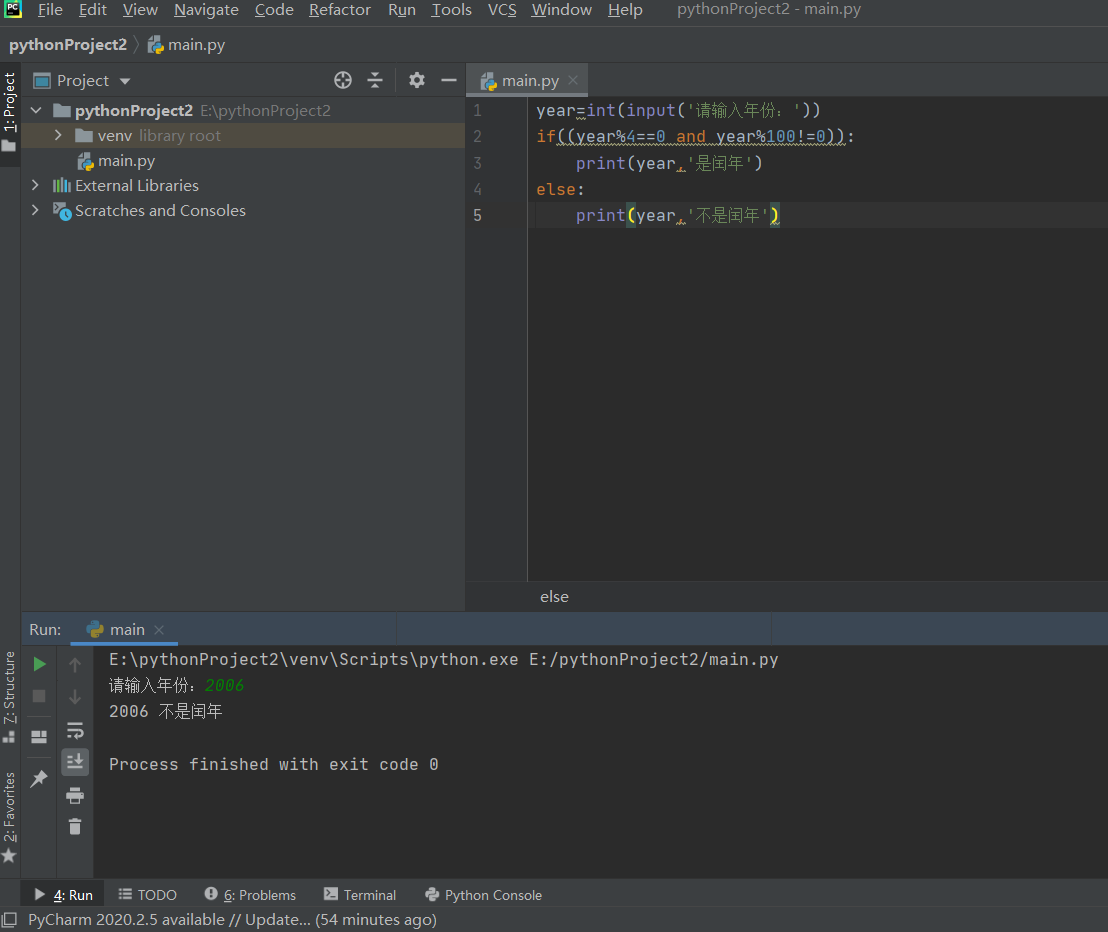三、预设一个0-9之间的整数，让用户猜一猜并输入所猜的数，如果大于预设的数，显示“太大”，小于预设的数，显示“太小"，如此循环，直至猜中该数，显示“恭喜 你猜中了”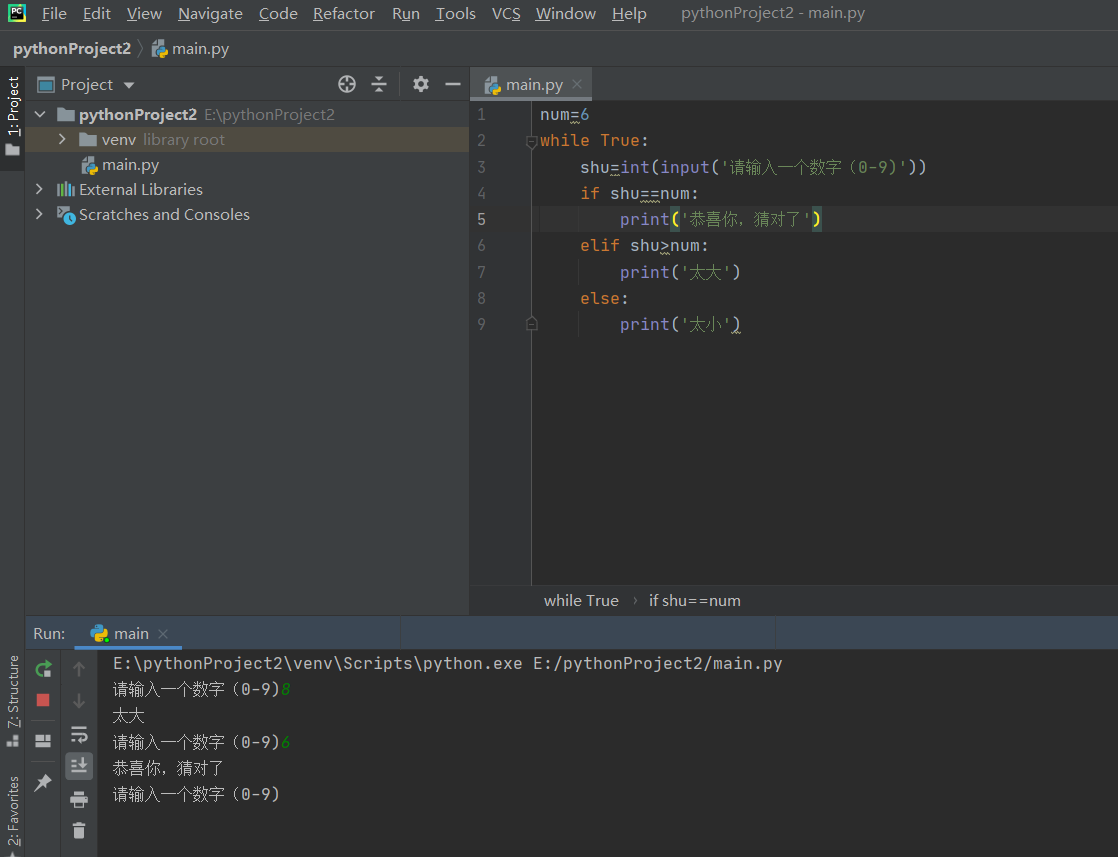四、求1-100间所有偶数的和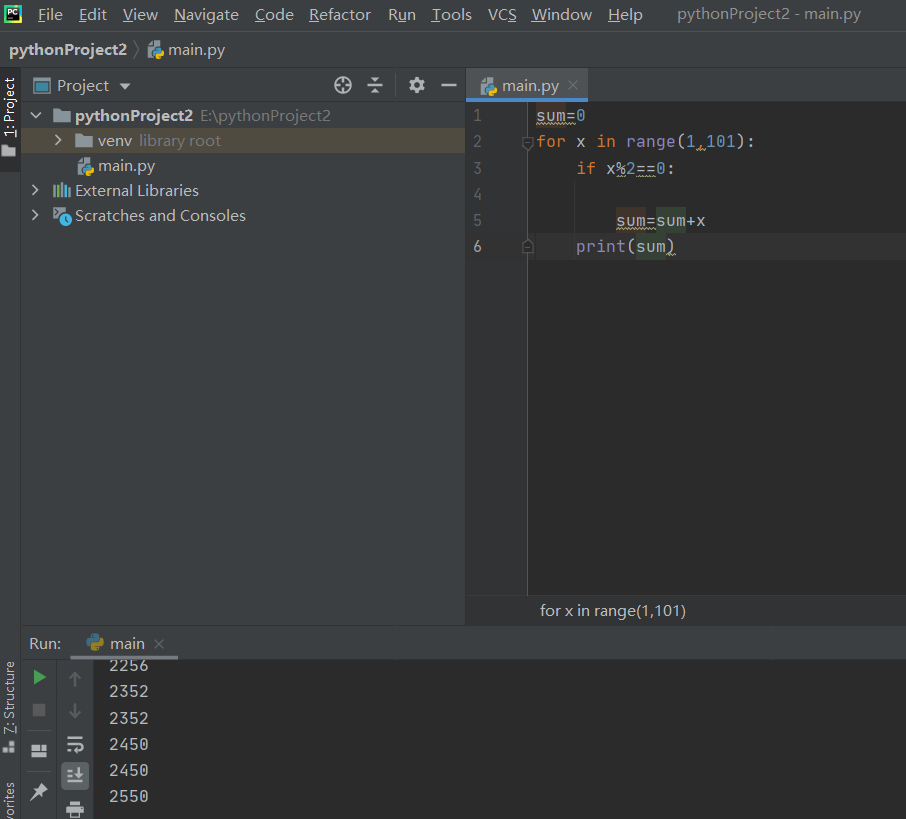五、输入三个数，比较大小输出最大的数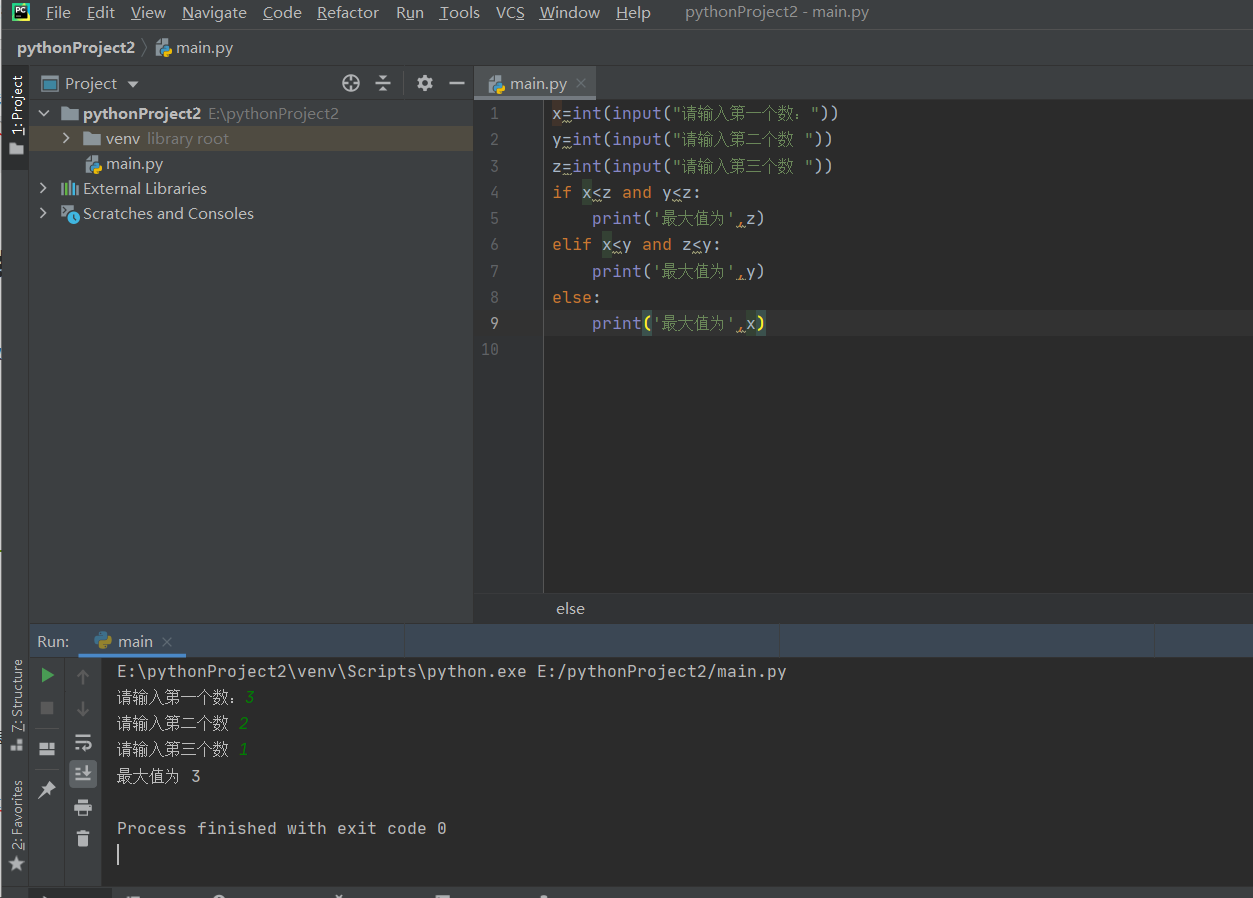总结
提示：这里对文章进行总结：
例如：以上就是今天要讲的内容，本文仅仅简单介绍了pandas的使用，而pandas提供了大量能使我们快速便捷地处理数据的函数和方法。


展开全文• 编程题 ...输出“Python语言简单易学” 第2章-1 计算 11+12+13+…+m 第2章-2 计算分段函数 第2章-3 阶梯电价 第2章-4 特殊a串数列求和 第2章-5 求奇数分之一序列前N项和 第2章-6 求...
• 第1章-1 从键盘输入两个数，求它们和并输出 a=int(input()) b=int(input()) print(a+b) 第1章-2 从键盘输入三个数到a,b,c中，按...第1章-3 输出“Python语言简单易学” s="Python语言简单易学" print(s.encode...
• Python程序设计入门32道基础编程题目与参考代码，该套题共有32道编程题，4个大题目类型：1.简单计算；2.字符串操作；3.流程控制；4.组合数据类型。每个类型每道题目对应参考代码分别以函数形式写在4个.py...
• 1、简述函数式编程 答：在函数式编程中，函数是基本单位，变量只是一个名称，而不是一个存储单元。...不过Python对匿名函数的支持有限，只有一些简单的情况下可以使用匿名函数。 def add(x,y): return x+y print(add
• 第1章-1 从键盘输入两个数，求它们和并输出 a=int(input()) b=int(input()) print(a+b) 第1章-2 从键盘输入三个数到a,b,c中，按...第1章-3 输出“Python语言简单易学” s="Python语言简单易学" print(s.encode...
• 问题描述：计算一道简单的题目。 源代码： #!/usr/bin/python # -*- coding: UTF-8 -*- if __name__ == '__main__': for i in range(5): n = 0 if i != 1: n += 1 if i == 3: n += 1 if i ==...数值计算
• 注意：我用的python2.7，大家如果用Python3.0以上版本，请记得在print()函数哦！如果因为版本问题评论，不做回复哦！！！ 1.题目：有1、2、3、4个数字，能组成多少个互不相同且无重复数字三位数？都是多少？ ...
• PTA基础编程题目集 之 Python解法（7-12） 7-12 两个数的简单计算器 (10分) 本题要求编写一个简单计算器程序，可根据输入运算符，对2个整数进行加、减、乘、除或求余运算。题目保证输入和输出均不超过整型范围。 ...
• 从零开始的Python学习 – 简单的练习题目录：从零开始的Python学习 -- 简单的练习题前言:顺序结构A+B problem苹果采购分支结构数的性质闰年判断Apple循环结构找到最小值分类平均阶乘之和前言:因为只看我的解释，很难...
• 实用标准文案 第一节课 Python 是什么类型的语言 Python 是脚本语言 脚本语言 (Scripting language) 是电脑编程语言 因此也能让开发者藉以编写出让电脑听命行事的程序 以简单的方式快速完成某些复杂的事情通常是创造...
• 简单的乐趣＃31：替换密码？【难度：2级】: 答案1： def is_substitution_cipher(s1, s2): return len(set(s1)) == len(set(s2)) == len(set(zip(s1, s2)))​ 答案2： import string def is_substitution_cipher...拼图
• 简单的乐趣＃59：反转对角线【难度：1级】: 答案1： def reverse_on_diagonals(matrix): copy = [ line[:] for line in matrix ] for i in range(len(matrix)): copy[i][i] = matrix[-1-i][-1-i] copy[i][-1-i.....拼图
• 简述函数式编程 在函数式编程中，函数是基本单位，变量只是一个名称，而不是一个存储单元。除了匿名函数外，Python还使用fliter(),map(),reduce(),apply()...匿名函数，也就是lambda函数，通常用在函数体比较简单的...
• 不要自卑，去提升实力 互联网行业谁技术牛谁是爹 ...放松一下，算一道简单的题目。 解题思路： > 代码： for i in range(5): n = 0 if i != 1: n += 1 if i == 3: n += 1 if i == 4: ..
• MITx 计算机科学和使用 Python 编程的介绍 ID 验证成就证书可在 讲座题目列表 第 1 讲 – 介绍： • 计算机做什么 • 计算思维 • 编程语言方面 • 基本机器架构 第 2 讲 – 程序核心元素： • 语言种类 • ......

# python简单的编程题目python 订阅